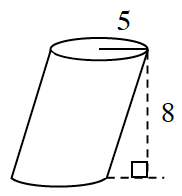### Home > PC3 > Chapter 12 > Lesson 12.1.5 > Problem12-79

12-79.

CAVALIERI’S PRINCIPLE

Bonaventura Cavalieri (1598-1647) was a mathematician who helped to develop calculus but is best remembered today for a principle named for him. Cavalieri’s Principle can be applied to calculate the volumes of solids that might be difficult to calculate otherwise.

1. Suppose you have a stack of $25$ pennies piled one on top of the other. You decide to slant the stack by sliding some of the pennies over. Does the volume of the $25$ pennies change because they are no longer stacked one on top of another?

2. Would the same thing be true of a stack of $15$ books if you slide the stack to the side or twist some of the books? What about a stack of $100$0 sheets of paper? Would the volumes change?

3.The idea of viewing solids as slices that can be moved around without affecting the volume is called Cavalieri's Principle. Use this principle to calculate the volume of the cylinder at right. Note that when the lateral faces of a prism or cylinder are not perpendicular to its base, the solid is referred to as an oblique cylinder or prism. How is the volume of the prism at right related to the one in problem 12-78?

Remember, you can check most of the homework answers in your textbook.